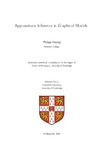## Approximate inference in graphical models

Hennig, Philipp
##### DOIThesis
##### Abstract

Probability theory provides a mathematically rigorous yet conceptually flexible calculus of uncertainty, allowing the construction of complex hierarchical models for real-world inference tasks. Unfortunately, exact inference in probabilistic models is often computationally expensive or even intractable. A close inspection in such situations often reveals that computational bottlenecks are confined to certain aspects of the model, which can be circumvented by approximations without having to sacrifice the model's interesting aspects. The conceptual framework of graphical models provides an elegant means of representing probabilistic models and deriving both exact and approximate inference algorithms in terms of local computations. This makes graphical models an ideal aid in the development of generalizable approximations. This thesis contains a brief introduction to approximate inference in graphical models (Chapter 2), followed by three extensive case studies in which approximate inference algorithms are developed for challenging applied inference problems. Chapter 3 derives the first probabilistic game tree search algorithm. Chapter 4 provides a novel expressive model for inference in psychometric questionnaires. Chapter 5 develops a model for the topics of large corpora of text documents, conditional on document metadata, with a focus on computational speed. In each case, graphical models help in two important ways: They first provide important structural insight into the problem; and then suggest practical approximations to the exact probabilistic solution.

##### Keywords
Applied mathematics, Computer science, Probability theory, Probabilistic inference, Graphical models, Approximate inference
##### Qualification
Doctor of Philosophy (PhD)
##### Awarding Institution
University of Cambridge